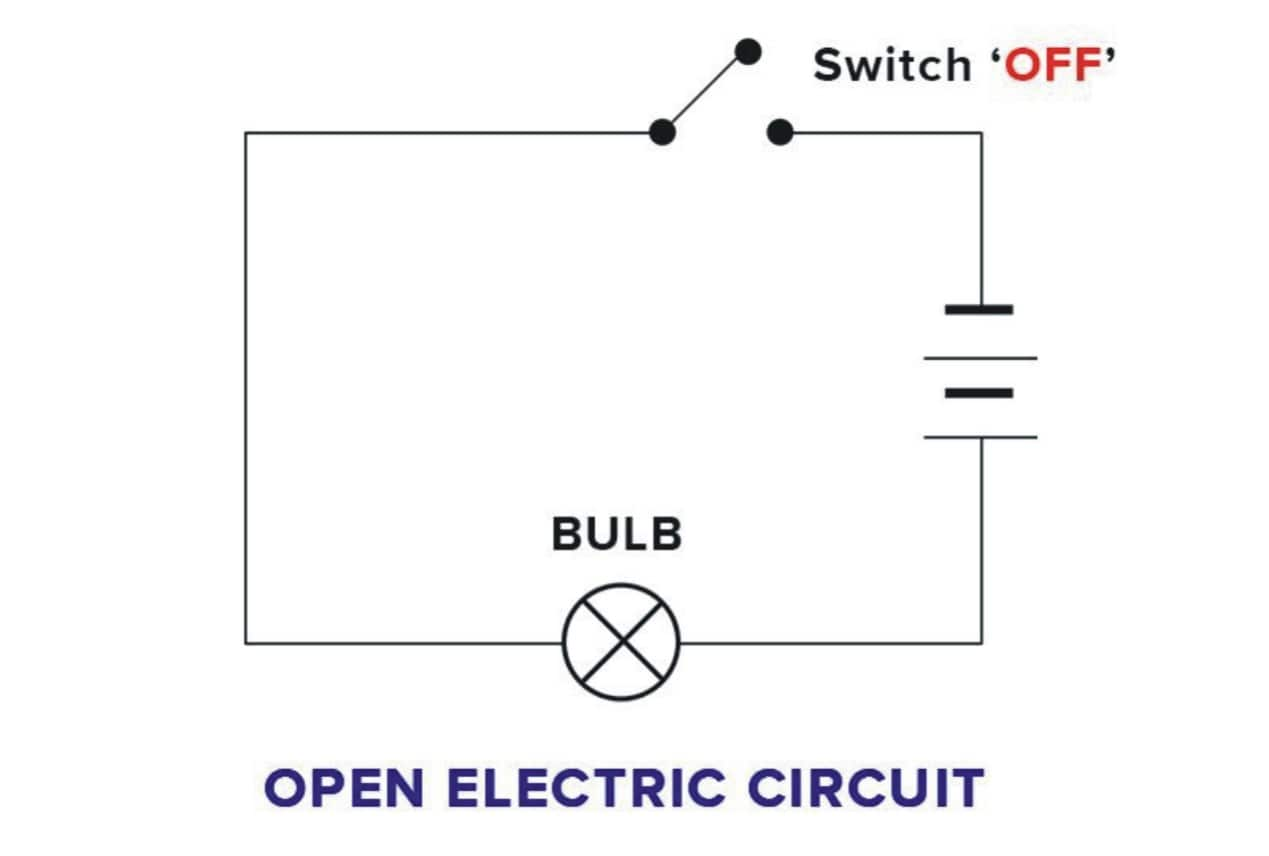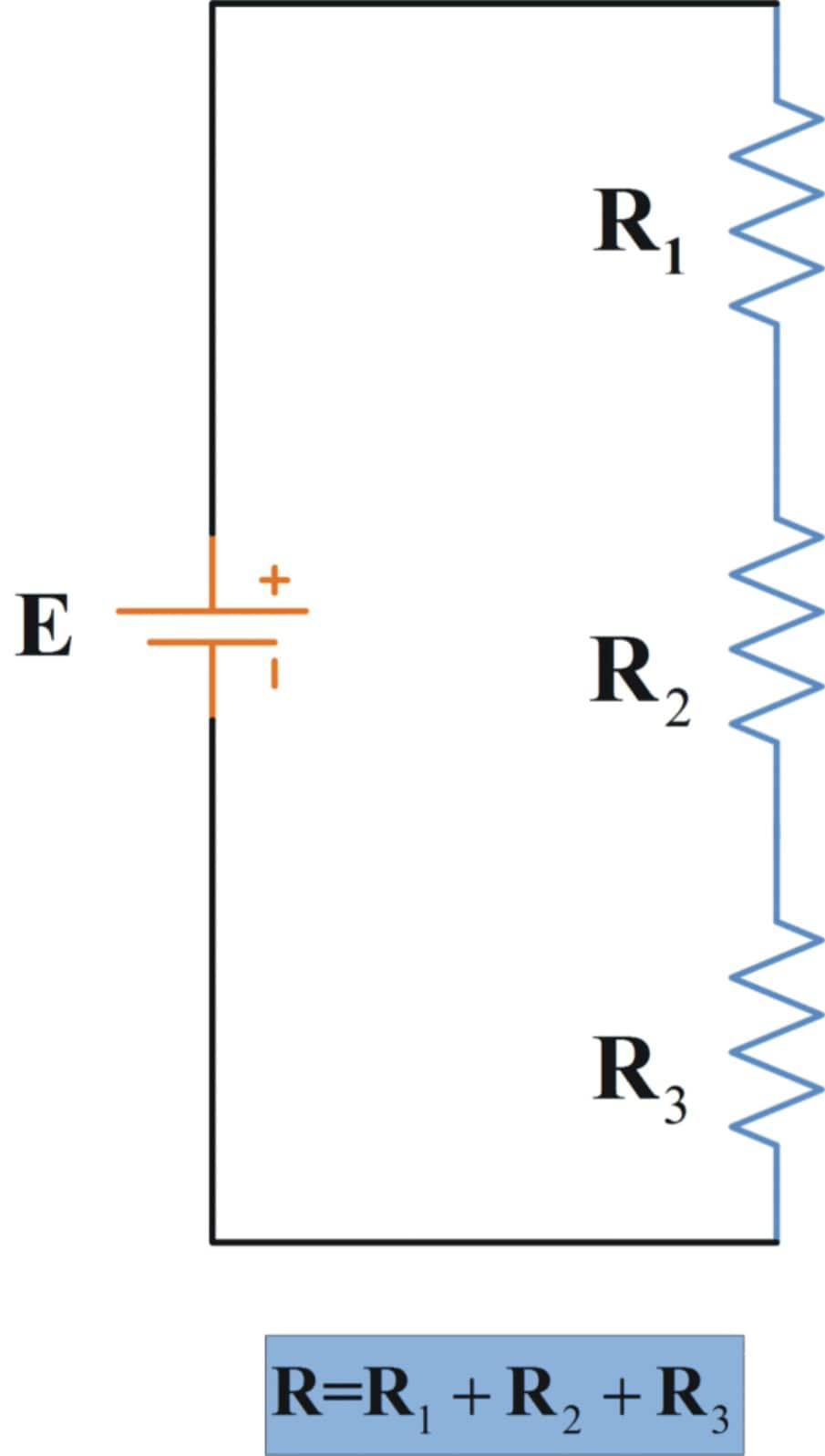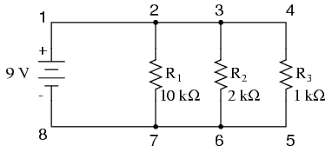# Difference Between Series and Parallel Circuits

## Series and Parallel Circuits - Formula and Examples

All electric devices need circuits to run smoothly and efficiently. Now, what are these circuits? Are they just electronic components put together and connected by wires? Well, almost. But a circuit is more than just that. Imagine, you can use a circuit to perform a task as simple as lighting a bulb and tasks as massive as getting a car to start. The placement of a component in the wrong area or the wrong terminal or even connecting the wrong ends can create a lot of issues. Hence, we should know all about the basics of circuits in order to master the trade.

In this particular article, we will learn more about the different types of circuits and how their differences and similarities. Read on, to find out more.

What is the circuit?

An electronic circuit can be defined as the complete course of electronic components such as conductors through which current can travel. These circuits provide a pathway for the flow of current. The main necessity of a circuit is that it should start and end at the same point. Or simply put, a circuit should form a loop. An electronic circuit is also called an electrical circuit.
A basic circuit consists of three components; they are the voltage source, load, and the conductive pathway.

Let's learn more about this.

1. VOLTAGE SOURCE

A voltage source provides the circuit with power or an energy source. It causes the current to flow. A typical example of a voltage source is a battery.

2. CONDUCTIVE PATHWAY

A conductive path basically provides a route for the current to flow through. The current flows through all the components right from the starting point back to the endpoint, which is also the starting point. It is essential that the conductive pathway forms a correct loop leading the voltage source from the negative terminal to the positive terminal.

3. LOAD

The load is the electronic component that consumes power. It does all the work in the circuit. It is said to be the heart of the circuit because, without a load, there is no point in building a circuit. It is practically useless.

A typical example of a load is a single light bulb. Light bulbs are used in simple circuits whereas, in complex bigger circuits, the load can be a combination of various other electronic components such as capacitors, resistors, transistors etc.
There are different types of circuits; the two basic ones are series and parallel circuits.SERIES CIRCUIT

A circuit is said to be in series when the same current flows through all the components connected in the circuit. The current has only one path. For example: If we take a light source, say fairy lights. This is basically a wire with multiple tiny bulbs connected in series to each other. If one bulb fuses the current stops at the fused bulb, all the bulbs after the fused bulb do not light up. The reason is that the circuit is connected in series. There is only one path for electricity. The amount of power is the same throughout all the components in the circuit. The speed or the rate of flow of electricity will never fluctuate throughout the entire circuit. The resistance of this circuit is the sum of all the individual resistances. The higher the number of resistors in the circuit, the higher the opposition, and hence the harder it is for current to flow.PARALLEL CIRCUIT

A circuit is said to be connected in parallel if it has multiple paths for electricity to flow through. Resistors and the sources will be connected between two sets of electrically common points. The electricity can flow in various directions, both horizontal as well as vertical. The components that are a part of a parallel circuit will have a constant voltage across all ends. The potential that the circuit carries will also be identical.

In a parallel circuit, there is always more than one resistor (bulb), and they are arranged in such a way that the electricity can travel through many paths. This means electricity (electrons) can move from one end of the cell through many branches to the other end of the cell.DIFFERENCES BETWEEN SERIES AND PARALLEL CIRCUITS

1. One of the significant differences is the formulae that are used for both series and parallel circuits to measure resistance.

For A Series Circuit
Total Resistance(R) = r1 (individual resistance)+r2+r3
For a Parallel Circuit
Total Resistance(R) = 1/r1 +1/r2 + 1/r3

2. Besides the difference in formula, the fact that makes series circuit different from the parallel circuit is that if one component in the series circuit breaks, then the resistor burns out, hence the circuit will not be complete. Whereas, in parallel circuits, however, the functioning of the other component

3. In a series circuit, the current at any point in the circuit is defined by one of in the most important and fundamental law of electricity, known as Ohm’s Law. To refresh your memory, Ohm’s Law states that I = V/R, where I stands for electrical current, V is the voltage supplied by the source and R represents the total resistance or the opposition to the flow of electric current of the circuit. Whereas in a parallel circuit, the current in each branch of the circuit is always inversely proportional to the resistance given by the resistor in each branch, and the total current is always equal to the sum of the currents in each individual branch.